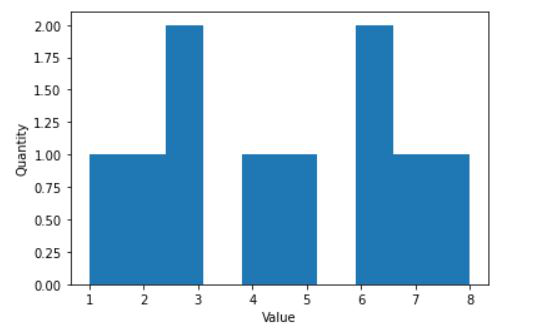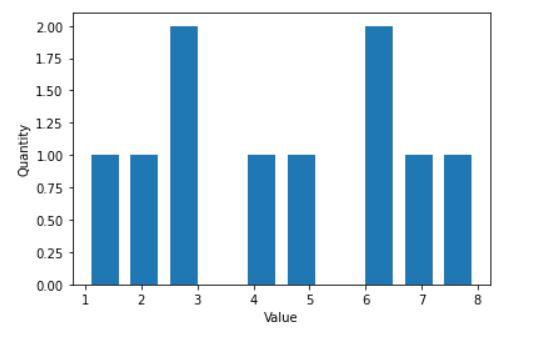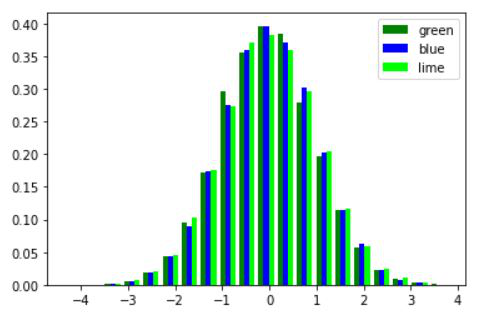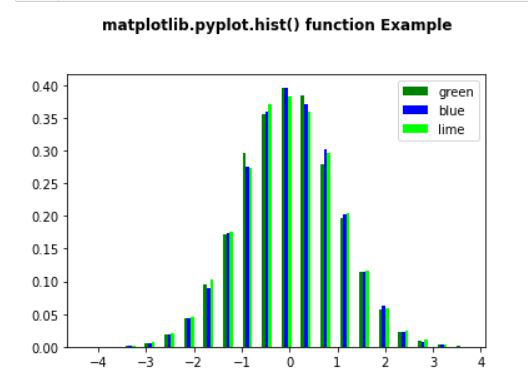Related Articles
Add space between histogram bars in Matplotlib
• Last Updated : 24 Jan, 2021

Matplotlib is a plotting library in Python programming language and it’s by default numerical mathematics extension of NumPy library in python language. While programming in python language we use the matplotlib library package for graph and histogram visualizations. But while plotting histogram using matplotlib in python, it lacks division or space between adjacent bars. This makes histograms very tedious to work with and it becomes very difficult to interpret. In this article, we will study how to add space between histogram bars in matplotlib.
Let’s understand with this example:

Example 1: Creating a simple plot.

## Python3

 `import` `matplotlib.pyplot as plt`` ` `values ``=` `[``1``, ``2``, ``3``, ``3``, ``4``, ``5``, ``6``, ``6``, ``7``, ``8``]`` ` `# Adjust the bar widths here``plt.hist(values)`` ` `plt.ylabel(``"Quantity"``)``plt.xlabel(``"Value"``)``plt.show()`

Output:Now we will add space between the histogram bars:

The space between bars can be added by using rwidth parameter inside the “plt.hist()” function. This value specifies the width of the bar with respect to its default width and the value of rwidth cannot be greater than 1.

## Python3

 `import` `matplotlib.pyplot as plt`` ` `values ``=` `[``1``, ``2``, ``3``, ``3``, ``4``, ``5``, ``6``, ``6``, ``7``, ``8``]`` ` `# Adjust the bar widths here``plt.hist(values, rwidth``=``0.7``)`` ` `plt.ylabel(``"Quantity"``)``plt.xlabel(``"Value"``)``plt.show()`

Output:Example 2: Creating a simple plot.

## Python3

 `# Implementation of matplotlib function ``import` `matplotlib ``import` `numpy as np ``import` `matplotlib.pyplot as plt ``     ` `np.random.seed(``10``*``*``7``) ``n_bins ``=` `20``x ``=` `np.random.randn(``10000``, ``3``) ``     ` `colors ``=` `[``'green'``, ``'blue'``, ``'lime'``] ``   ` `plt.hist(x, n_bins, density ``=` `True``,  ``         ``histtype ``=``'bar'``, ``         ``color ``=` `colors, ``         ``label ``=` `colors) ``   ` `plt.legend(prop ``=``{``'size'``: ``10``})   ``plt.show()`

Output:With adding space:

## Python3

 `# Implementation of matplotlib function ``import` `matplotlib ``import` `numpy as np ``import` `matplotlib.pyplot as plt ``     ` `np.random.seed(``10``*``*``7``) ``n_bins ``=` `20``x ``=` `np.random.randn(``10000``, ``3``) ``     ` `colors ``=` `[``'green'``, ``'blue'``, ``'lime'``] ``   ` `plt.hist(x, n_bins, density ``=` `True``,  ``         ``histtype ``=``'bar'``, ``         ``color ``=` `colors, ``         ``label ``=` `colors,``         ``rwidth ``=` `0.5``) ``   ` `plt.legend(prop ``=``{``'size'``: ``10``}) ``   ` `plt.title(``'matplotlib.pyplot.hist() function Example\n\n'``, ``          ``fontweight ``=``"bold"``) ``   ` `plt.show()`

Output:Attention geek! Strengthen your foundations with the Python Programming Foundation Course and learn the basics.

To begin with, your interview preparations Enhance your Data Structures concepts with the Python DS Course.

My Personal Notes arrow_drop_up